2020-03-14 22:55

# C语言 求助大佬这样的数字怎么输出？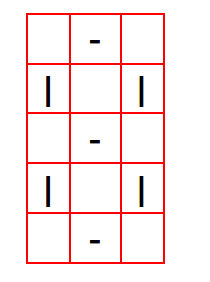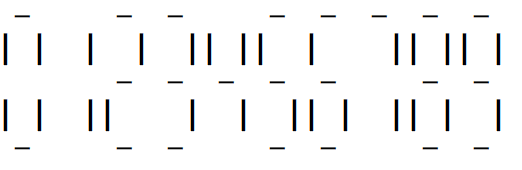0123456789

• 点赞
• 写回答
• 关注问题
• 收藏
• 邀请回答

#### 1条回答默认 最新

•智者知已应修善业 2020-03-15 11:56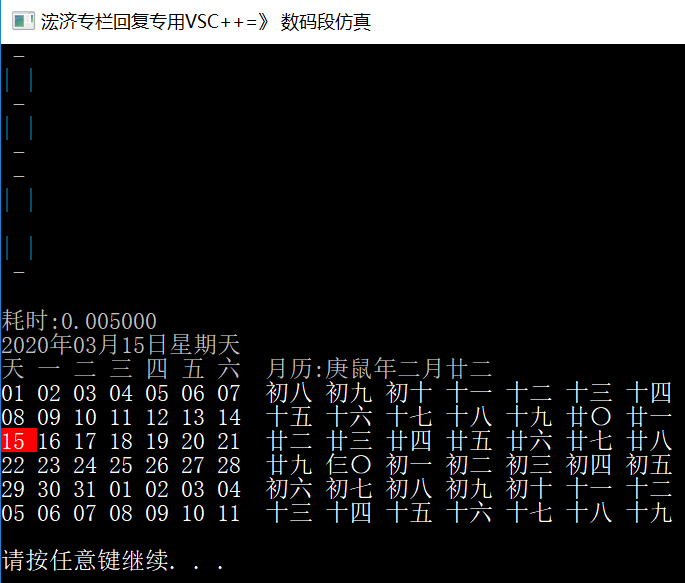``````    string a = "   \n", a1 = " - \n", a2 = "| |\n", a3 = "|  \n", a4 = "  |\n";
cout << a1 << a2 << a1 << a2 << a1;
cout << a1 << a2 << a << a2 << a1;
``````

由此推导，本题也就算是个逻辑的组合：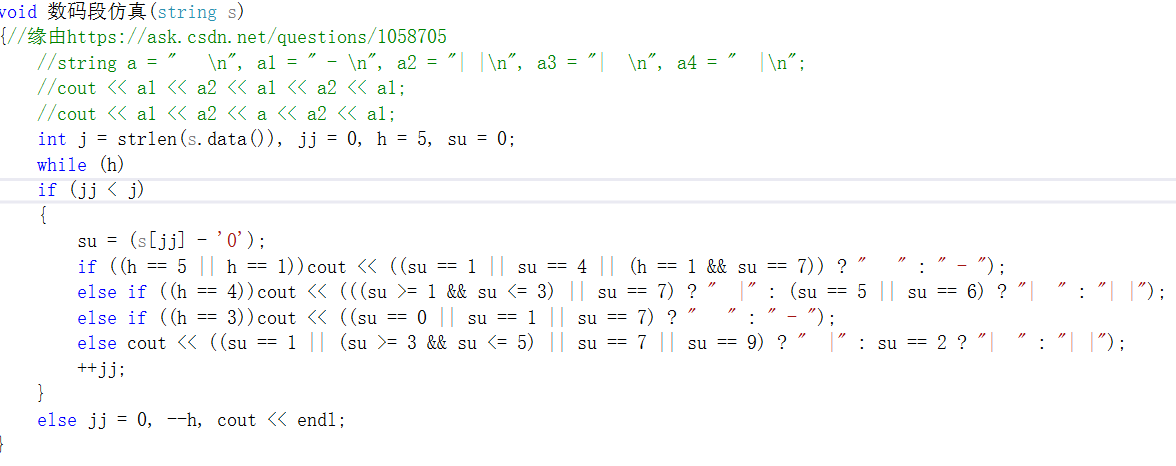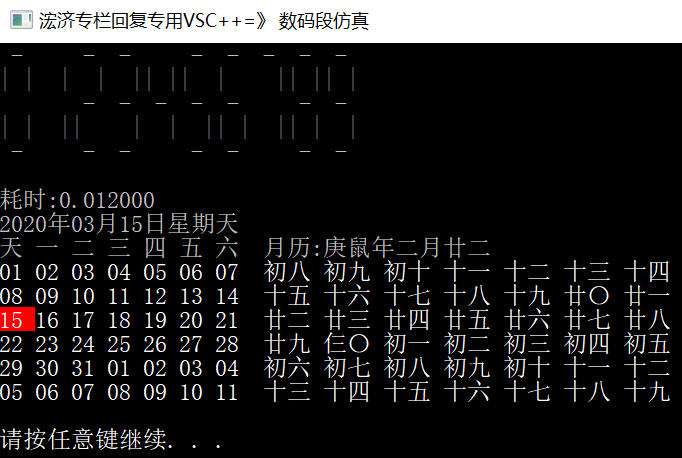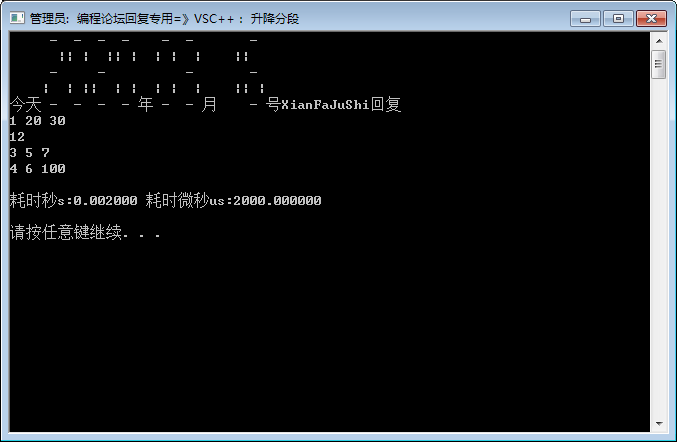点赞 评论